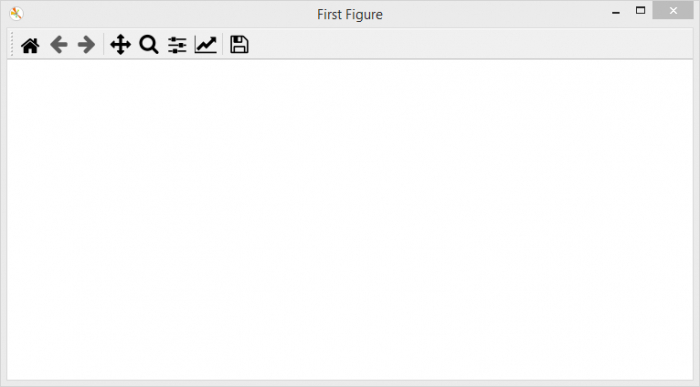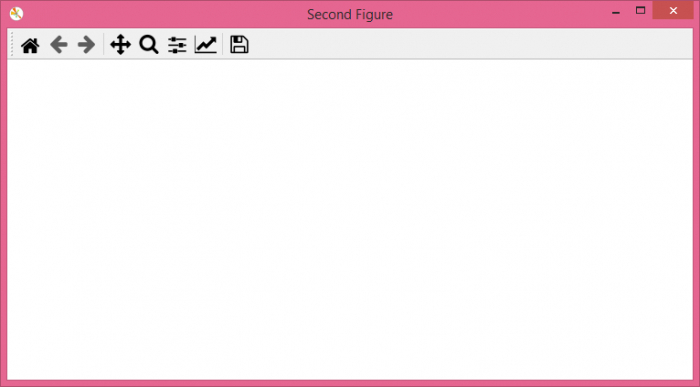# Flushing all current figures in matplotlib

To flush all current figures in matplotlib, use close('all') method.

## Steps

• Set the figure size and adjust the padding between and around the subplots.

• Create a figure with the title "First Figure".

• Create another figure with the title "Second Figure".

• To close all figures, use close('all').

• To display the figure, use show() method.

## Example

from matplotlib import pyplot as plt

plt.rcParams["figure.figsize"] = [7.50, 3.50]
plt.rcParams["figure.autolayout"] = True

plt.figure("First Figure")
plt.figure("Second Figure")

# plt.close('all')

plt.show()

## Output

Notice that we have commented the line −

plt.close('all')


Hence, it will display two figures −Uncomment the line plt.close('all') and run the code again. It will flush all the current figures.